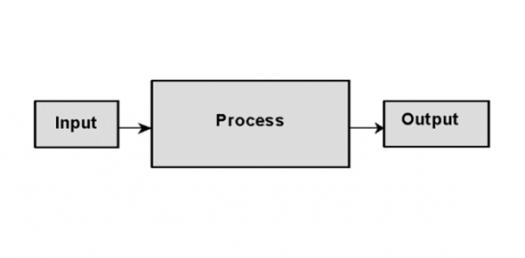# Basic Input Output System(BIOS) Quizzes

70 Questions | Total Attempts: 1179SettingsAll are related to Basic Input Output System(BIOS).

• 1.
What is the first job the Basic Input Output System(BIOS) does at start-up?
• A.

Run memory byte count

• B.

Test for parity errors

• C.

• D.

Run the power-on-self-test

• 2.
Which original component was very susceptible to damage from electrostatic discharge?
• A.

• B.

Basic Input Output System

• C.

Complimentary metal-oxide semiconductor(CMOS)

• D.

EEPROM

• 3.
Which component uses a lithium battery to maintain computer settings when power is removed?
• A.

ROM

• B.

BIOS

• C.

CMOS

• D.

EEPROM

• 4.
What is considered as nonvolatile permanent memory?
• A.

ROM

• B.

BIOS

• C.

CMOS

• D.

EEPROM

• 5.
What is a form of firmware that contains the computer's startup instructions?
• A.

BIOS

• B.

CMOS

• C.

Cache

• D.

EEPROM

• 6.
What static RAM(SRAM) uses special applications in a CPU?
• A.

BIOS

• B.

CMOS

• C.

Cache

• D.

EEPROM

• 7.
On most modern computers, which has two or three levels of memory?
• A.

CMOS

• B.

Cache

• C.

DDRAM

• D.

EEPROM

• 8.
Actively sampling the status of an external device by a client program is known as
• A.

Polling

• B.

Signaling

• C.

Processing

• D.

• 9.
What is defined as an asynchronous signal from a computer hardware device indicating the need for attention from a central processing unit(CPU) or a synchronous event?
• A.

Cache

• B.

Buffer

• C.

Interface

• D.

Interrupt

• 10.
What interrupt request(IRQ) line number is normally used for the floppy disk controller?
• A.

2

• B.

4

• C.

6

• D.

8

• 11.
Which term describes that data is whole or complete?
• A.

Driver

• B.

Parity bit

• C.

Data Integrity

• D.

Error Correction

• 12.
What is considered as a technique or method of checking data integrity?
• A.

Asynchronous

• B.

Parity bit

• C.

Interrupt

• D.

Fault

• 13.
Which is a very simple example of an error detecting code?
• A.

Interrupt

• B.

Parity bit

• C.

Asynchronous

• D.

Data Integrity

• 14.
What component initializes communication with all hardware devices and sends a message if a keyboard or mouse is not found?
• A.

ROM

• B.

BIOS

• C.

CMOS

• D.

EEPROM

• 15.
Which is a small, low-level program used by the computer operating system to interact with hardware devices?
• A.

BIOS

• B.

Driver

• C.

Parity

• D.

Interrupt

• 16.
Static electricity remains intact until it is
• A.

Absorbed

• B.

Dissolved

• C.

Discharged

• D.

Neutralized

• 17.
Under what class of electrostatic discharge(ESD) would an item fall if it was sensitive to 900 volts?
• A.

I

• B.

II

• C.

III

• D.

IV

• 18.
Electrostatic discharge(ESD) items sensitive to more than 4,000 but less than 15,000 volts fall into category
• A.

I

• B.

II

• C.

III

• D.

IV

• 19.
What measure would you take to prevent the build up of static electricity?
• A.

Ground the ESDS device

• B.

Check the relative humidity level

• C.

Disconnect the circuit from the device

• D.

Turn the power switch to the off position

• 20.
What is the first step you must take when either installing or removing an electrostatic discharge sensitive(ESDS) device?
• A.

Ground the ESDS device

• B.

Check the relative humidity level

• C.

Disconnect the circuit from the device

• D.

Turn the power switch to the off position

• 21.
The process of collecting and analyzing data to determine the cause of an operational failure and how to prevent it from recurring is called?
• A.

Fault isolation

• B.

Event evaluation

• C.

Process investigation

• D.

Analytical consideration

• 22.
In which troubleshooting step would you determine which conditions are present?
• A.

Define the problem

• B.

Isolate the problem

• C.

Resolve the problem

• D.

Confirm the resolution

• 23.
In which troubleshooting step would you confirm a dilemma exists?
• A.

Define the problem

• B.

Isolate the problem

• C.

Resolve the problem

• D.

Confirm the resolution

• 24.
In which troubleshooting step would you identify likely causes and eliminate unlikely causes?
• A.

Define the problem

• B.

Isolate the problem

• C.

Resolve the problem

• D.

Confirm the resolution

• 25.
In which troubleshooting step would you review the case history and assure no steps were missed?
• A.

Define the problem

• B.

Isolate the problem

• C.

Resolve the problem

• D.

Confirm the resolution

Related TopicsBack to top